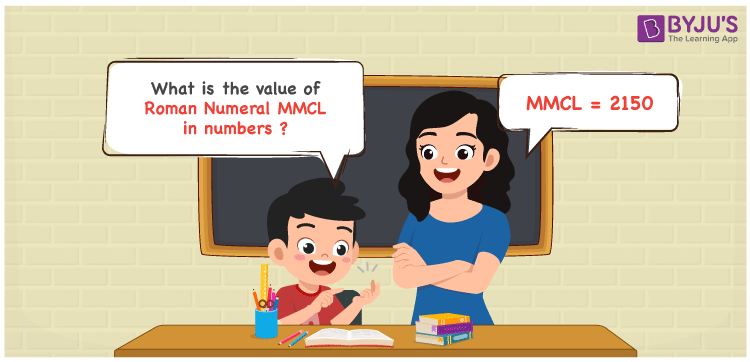Checkout JEE MAINS 2022 Question Paper Analysis : Checkout JEE MAINS 2022 Question Paper Analysis :

# MMCL Roman Numerals

MMCL Roman Numerals is 2150. In this article, MMCL is split and the respective number for each English alphabet is indicated. With the help of the addition operation in Maths, the numeric form of the roman numerals MMCL can be obtained effortlessly. Roman numerals articles possess the details important from the exam perspective. Therefore, MMCL can be indicated as 2150 in numbers.

 Number Roman Numeral 2150 MMCL## How to Write MMCL Roman Numerals in Numbers?

MMCL is expanded and converted to numbers in an interesting way to improve the problem solving abilities among students.

MMCL = M + M + C + L

MMCL = 1000 + 1000 + 100 + 50

MMCL = 2150

## Video Lesson on Roman Numerals## Frequently Asked Questions on MMCL Roman Numerals

### Why is the roman numeral of 2150 written as MMCL?

We know that

MMCL = M + M + C + L

MMCL = 1000 + 1000 + 100 + 50

MMCL = 2150

### Determine the value of 2200 – 50.

We know that

2200 – 50 = 2150

Hence, the value of 2200 – 50 is 2150 which is written as MMCL.

### What is the remainder we obtain when MMCL is divided by XII?

We know that

MMCL = 2150

XII = 12

So the remainder we obtain when MMCL is divided by XII is II.